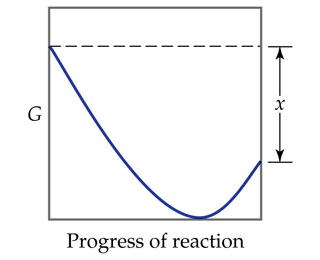# Problem: The accompanying diagram shows how the free energy, G, changes during a hypothetical reaction A(g) +B(g) →  C(g). On the left are pure reactants A and B, each at 1 atm, and on the right is the pure product, C, also at 1 atm. Indicate whether each of the following statements is true or false.The minimum of the graph corresponds to the equilibrium mixture of reactants and products for this reaction.

###### FREE Expert Solution

We’re asked to indicate whether the following statement is true or false from the given plot.

We're given a the plot of Gibb's Energy, G as the hypothetical reaction A (g) + B (g) → C (g) proceeds.

Recall that the change in Gibbs free energy ΔG, tells us whether a reaction is spontaneous or non spontaneous

$\overline{){\mathbf{∆}}{\mathbf{G}}{\mathbf{=}}{\mathbf{G}}{{\mathbf{°}}}_{\mathbf{f}\mathbf{,}\mathbf{products}}{\mathbf{-}}{\mathbf{G}}{{\mathbf{°}}}_{\mathbf{f}\mathbf{,}\mathbf{reactants}}}$

• ΔG < 0 or ΔG = (–); the reaction is spontaneous

• ΔG = 0; the reaction is at equilibrium

• ΔG > 0 or ΔG = (+); the reaction is non-spontaneous

From the plot, we can see that the Gibbs energy, G decreases as the reaction proceeds.

Analyzing the statement:

The minimum of the graph corresponds to the equilibrium mixture of reactants and products for this reaction.

100% (127 ratings)###### Problem Details

The accompanying diagram shows how the free energy, G, changes during a hypothetical reaction A(g) +B(g) →  C(g). On the left are pure reactants A and B, each at 1 atm, and on the right is the pure product, C, also at 1 atm. Indicate whether each of the following statements is true or false.The minimum of the graph corresponds to the equilibrium mixture of reactants and products for this reaction.